# Please answer me in detail. Thank you. Question 9 Suppose that a monopolist faces a demand curve given by P 120-2Q. A m...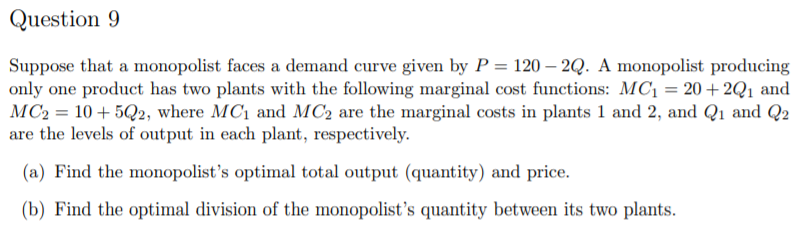Question 9 Suppose that a monopolist faces a demand curve given by P 120-2Q. A monopolist producing only one product has two plants with the following marginal cost functions: MC1 20+2Q1 and MC2-10+502, where MCi and MC2 are the marginal costs in plants 1 and 2, and Q1 and Q2 are the levels of output in each plant, respectively. (a) Find the monopolist's optimal total output (quantity) and price. b) Find the optimal division of the monopolist's quantity between its two plants.

P = 120 - 2Q

(a)

Total revenue (TR) = P x Q = 120Q - 2Q2

Marginal revenue (MR) = dTR/dQ = 120 - 4Q

MC1 = 20 + 2Q1, therefore Q1 = (MC1 - 20) / 2 = 0.5MC1 - 10

MC2 = 10 + 5Q2, therefore Q2 = (MC2 - 10) / 5 = 0.2MC2 - 2

As Q = Q1 + Q2,

Q = 0.5MC1 - 10 + 0.2MC2 - 2

Q = 0.5MC1 + 0.2MC2 - 12

Equating MC1 and MC2 as MC,

Q = 0.5MC + 0.2MC - 12

Q = 0.7MC - 12

0.7MC = Q + 12

MC = (Q + 12) / 0.7

Profit-maximizing condition is: MR = MC.

120 - 4Q = (Q + 12) / 0.7

84 - 2.8Q = Q + 12

3.8Q = 72

Q = 18.95

P = 120 - (2 x 18.95) = 120 - 37.9 = 82.1

(b)

MC = (Q + 12) / 0.7 = (18.95 + 12) / 0.7 = 30.95 / 0.7 = 44.21

Q1 = 0.5MC - 10 = (0.5 x 44.21) - 10 = 22.11 - 10 = 12.11

Q2 = 0.2MC - 2 = (0.2 x 44.21) - 2 = 8.84 - 2 = 6.84

##### Add Answer of: Please answer me in detail. Thank you. Question 9 Suppose that a monopolist faces a demand curve given by P 120-2Q. A m...
Similar Homework Help Questions
• ### 5. A monopolist faces a demand curve P = 60 – 2Q and initially faces a...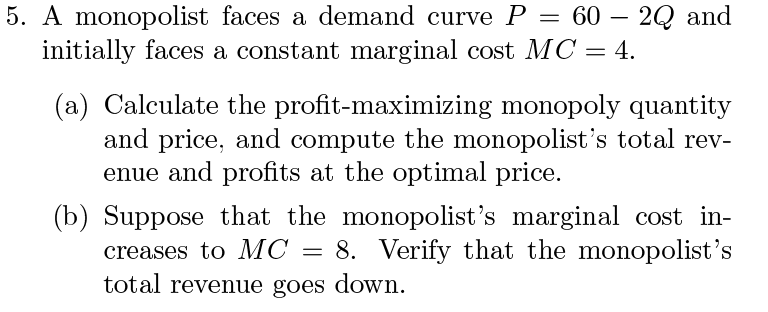5. A monopolist faces a demand curve P = 60 – 2Q and initially faces a constant marginal cost MC = 4. (a) Calculate the profit-maximizing monopoly quantity and price, and compute the monopolist's total rev- enue and profits at the optimal price. (b) Suppose that the monopolist's marginal cost in- creases to MC = 8. Verify that the monopolist's total revenue goes down. (c) Suppose that all firms in a perfectly competitive equilibrium had a constant marginal cost MC...

• ### Show work please A monopolist's inverse demand function is P= 150 – 3Q. The company produces...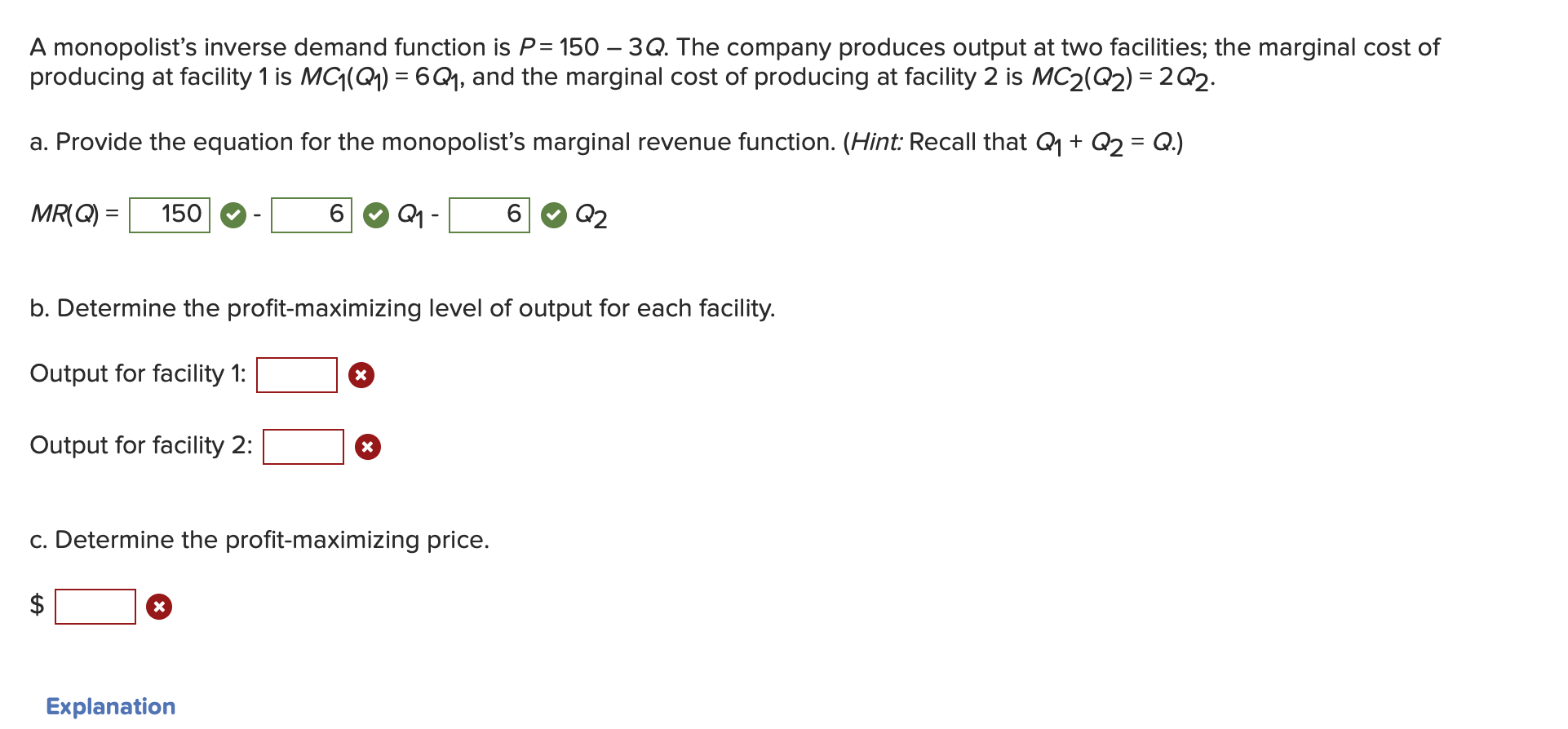Show work please A monopolist's inverse demand function is P= 150 – 3Q. The company produces output at two facilities; the marginal cost of producing at facility 1 is MC1(Q1) = 6Q1, and the marginal cost of producing at facility 2 is MC2(Q2) = 2Q2: a. Provide the equation for the monopolist's marginal revenue function. (Hint: Recall that Q1 + Q2 = Q.) MR(Q) = 150-C6 Q4-06 Q2 b. Determine the profit-maximizing level of output for each facility. Output for...

• ### Market demand is P (Q) = 40 − Q. A multi-plant monopolist operates two plants, with...

Market demand is P (Q) = 40 − Q. A multi-plant monopolist operates two plants, with average cost functions AC1(q1) = 8 and AC2(q2) = 1 + 0.25q2. a) Find the profit-maximizing price, total quantity and of output produced at each plant. b) Is this multi-plant monopolist allocatively efficient? Explain carefully. Do not calculate socially efficient output.

• ### A monopolist faces inverse demand P = on TC(Q) = cQ. (a) Find the optimal price,...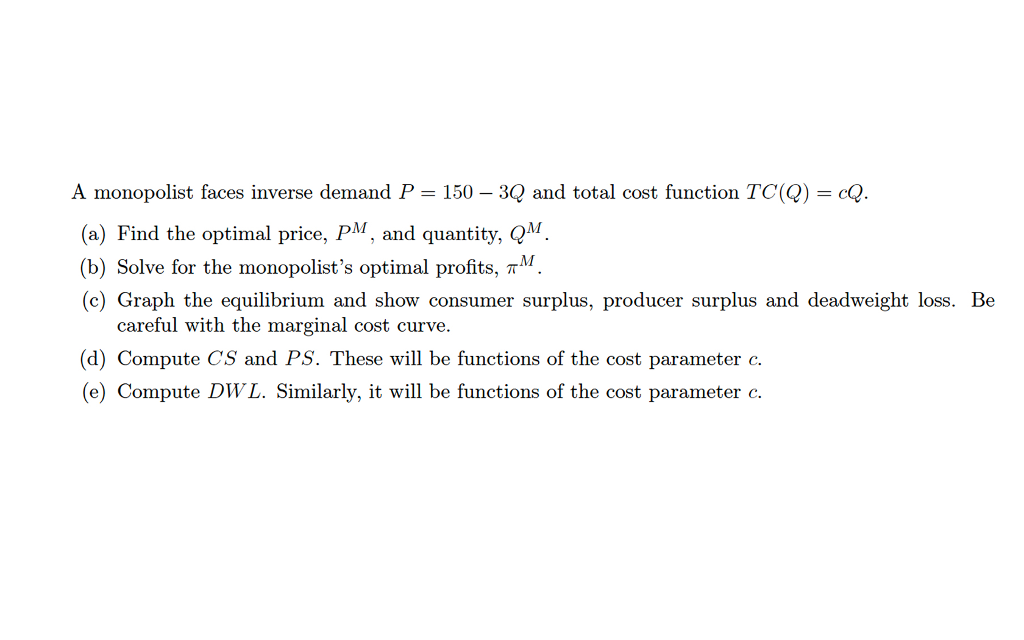A monopolist faces inverse demand P = on TC(Q) = cQ. (a) Find the optimal price, P, and quantity, QM (b) Solve for the monopolist's optimal profits, TM (c) Graph the equilibrium and show consumer surplus, producer surplus and deadweight loss. Be 150 -3Q and total cost functi careful with the marginal cost curve. (d) Compute CS and PS. These will be functions of the cost parameter c. (e) Compute DWL. Similarly, it will be functions of the cost parameter...

• ### 4 A monopolist faces a market demand curve given by Q-70-P a. If the monopolist can produce at co...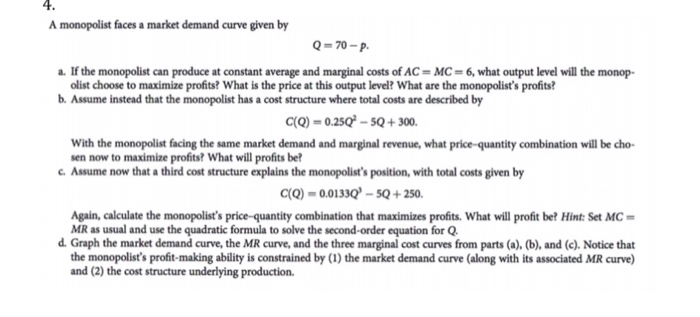4 A monopolist faces a market demand curve given by Q-70-P a. If the monopolist can produce at constant average and marginal costs ofAC-MC-6, what output level will the monop- olist choose to maximize profits? What is the price at this output level? What are the monopolist's profits? b. Assume instead that the monopolist has a cost structure where total costs are described by C(Q)-.250-sQ+300. With the monopolist facing the same market demand and marginal revenue, what price-quantity combination will...

• ### Microeconomics  A monopolist with cost function c(Q) demand functions are given by. faces a consumer...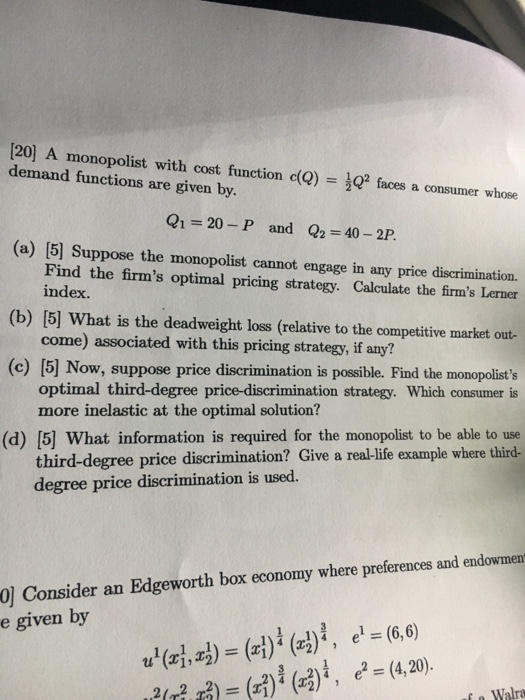Microeconomics  A monopolist with cost function c(Q) demand functions are given by. faces a consumer whose Q1=20-P and Q2-40-2P. (a)  Suppose the monopolist cannot engage in any price discrimination. Find the firm's optimal pricing strategy. Calculate the firm's Lerner index. come) associated with this pricing strategy, if any? optimal third-degree price-discrimination strategy. Which consumer is (b)  What is the deadweight loss (relative to the competitive market out- (c)  Now, suppose price discrimination is possible. Find the...

• ### A natural monopolist faces the following demand curve: P = 202 - 5Q, its total cost is given by: ...

A natural monopolist faces the following demand curve: P = 202 - 5Q, its total cost is given by: TC = 720 + 2Q (marginal cost is the slope of total cost). (a) If the government regulates the monopolist to charge a socially optimal price, what price will it charge and how many units will it sell? How much are the profit, consumer surplus and producer surplus? (b) If it is not a regulated monopolist, what is its profit maximizing...

• ### A two product monopolist faces the demand and cost functions as below: Q1=40-2(P1)-(P2) Q2=35-(P1)-(P2) C=(Q1)^2+2(Q2)^2+10 a) Find the profit maximizing levels of output and the price charged for each product

A two product monopolist faces the demand and cost functions as below:Q1=40-2(P1)-(P2) Q2=35-(P1)-(P2) C=(Q1)^2+2(Q2)^2+10a) Find the profit maximizing levels of output and the price charged for each product.

• ### You are a monopolist facing inverse demand for your product given by P = 120 -...

You are a monopolist facing inverse demand for your product given by P = 120 - 2Q and you have constant marginal cost given by MC = 30 A. Assume you can charge 2 different prices based on the quantity purchased. What are the producers surplus maximizing levels of these prices? B. Show graphically how much more producer's surplus you make by setting 2 prices instead of 1 in this market.

• ### 1. Suppose that demand is given by P=100-Q, marginal revenue is MR=100-2Q, and marginal cost (and...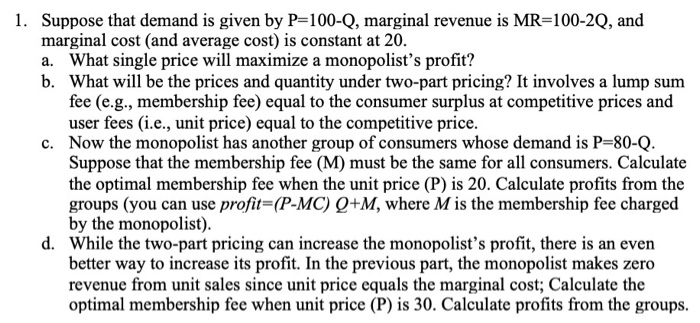1. Suppose that demand is given by P=100-Q, marginal revenue is MR=100-2Q, and marginal cost (and average cost) is constant at 20. a. What single price will maximize a monopolist's profit? b. What will be the prices and quantity under two-part pricing? It involves a lump sum fee (e.g., membership fee) equal to the consumer surplus at competitive prices and user fees (i.e., unit price) equal to the competitive price. c. Now the monopolist has another group of consumers whose...

Need Online Homework Help?